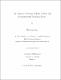## On Hadwiger covering problem in five- and six-dimensional Euclidean spacesWe denote by $H_n$ the minimum number such that any convex body in $\mathbb{R}^{n}$ can be covered by $H_n$ of its smaller homothets. Considering an $n$-dimensional cube, one can easily see that $H_n\geqslant2^{n}$. It is a well-known conjecture that $H_n= 2^{n}$ for all $n\geqslant 3$. The main result of this thesis is the inequalities $H_5\leqslant 1002$ and $H_6\leqslant 14140$. The previously known upper bounds were $H_5\leqslant 1091$ and $H_6\leqslant 15373$. Specifically, we apply certain generalizations of an approach by Papadoperakis, which essentially reduces the problem to the study of covering of $(n-2)$-dimensional faces of an $n$-dimensional cube by parallelepipeds of a particular form. A step in the construction of the required covering uses computer assistance. We also study limitations of this technique and establish some lower bounds on performance of this method.